Equity Valuation: Concepts and Basic Tools | IFT World
IFT Notes for Level I CFA® Program
IFT Notes for Level I CFA® Program

# Part 2

## 6. Preferred Stock Valuation

For a non-callable, non-convertible perpetual preferred share paying a level dividend and assuming a constant required rate of return, the value is given by the equation below: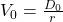where: V0 = present value of the perpetuity; D0 = dividend and r = rate of return

Example

A $100 par value, non-callable, non-convertible perpetual preferred stock pays a 5% dividend. The discount rate is 8%. Calculate the intrinsic value of the preferred share. Solution: Expected annual dividend = 0.05 x 100 = 5 Value of the preferred share = 5.00/0.08 = 62.50 Other types of preferred shares to consider are: • Shares which mature on a given date: In the earlier example, instead of being a perpetual share, assume the share matured after four years. To calculate the value of this share, calculate the present value of the four dividends with the last one paid at the end of 4thyear at the required rate of 8%. Input these values in your financial calculator: N = 4; I = 8; PMT = 5; FV = 100; PV =? Present value of this share = 90.06. • Callable (redeemable) shares: These shares are callable by the issuer at some point before maturity. Assuming all the conditions are the same as the shares which mature on a given date, will investors pay 90.06, less or more for this share? They will pay less for this share as investors stand the risk of the issuer calling the share when it trades above the par value. • Shares with retraction option (putable shares): Here, the holder of the preferred stock has an option to sell the share to the issuer at a specified price before the maturity date. Unlike callable shares, putable shares will trade at a value above 90.06 as the put option is valuable to investors. If the share trades below the par value, investors can sell it back to the issuer. ## 7. The Gordon Growth Model One of the disadvantages of the dividend discount model is that it is difficult to accurately estimate the amount of dividends for a long period of time. The Gordon growth model simplifies this by assuming that dividends grow indefinitely at a constant rate; it is also called the constant-growth dividend discount model. According to this model, the intrinsic value of a security can be calculated as: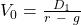where: g = dividend growth rate = b * ROE b = earnings retention rate = (1- dividend payout ratio) ROE = return on equity In the equation above, if the growth rate is zero, then the equation reduces to the present value of a perpetuity. Assumptions of the Gordon Growth model: • Dividends are the correct metric to use for valuation purposes. Dividends are a reflection of a company’s earnings. • Dividend growth rate is perpetual. • Required rate of return is constant throughout the life of the security. • Dividend growth rate < required rate of return. When is it not appropriate to use the Gordon Growth Model? • If the company is currently not paying a dividend as it may reinvest earnings in attractive opportunities. • If the company is not profitable enough currently to pay a dividend. An analyst may still use the model by assuming that the company will pay a dividend in the future. What happens to the value if dividend value is increased? Let us look at the formula again.When dividend increases, numerator increases. If the payout ratio increases, retention rate decreases and value of g decreases. If g decreases, the denominator increases. As a result, the impact on value, if dividend is increased cannot be determined with certainty. Example Estimate the intrinsic value of a stock given the following data: Beta =1.5; RFR = 3%; market risk premium = 5%; dividend just paid =$1.00; dividend payout ratio = 0.4; return on equity = 15%.

Solution: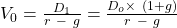Note: the values of r, g and expected dividend are not given. So, first calculate these values.

r = RFR + Beta x market risk premium = 3+ 1.5 x 5 = 10.5%

g = b x ROE = (1 – 0.4) x 0.15 = 0.09

Applying the Gordon growth model, V0== 72.67

Example

A company does not currently pay dividend but is expected to begin to do so in 4 years. The first dividend is expected to be $2.00 and to be received at the end of year 4. The dividend is expected to grow at 5% into perpetuity. The required return is 10%. What is the estimated current intrinsic value? Solution: To calculate the intrinsic value, first calculate the value of dividend at the end of period 3 and then discount it to t=0 using the Gordon growth model. V3 =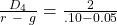= 40 V== 30.05 Instructor’s Note: Do not forget to discount 40 to the present value. The undiscounted value is commonly presented as one of the answer options as a trap. ## 8. Multistage Dividend Discount Models It is an ideal situation to assume that all companies grow at a constant rate indefinitely and pay a constant dividend; the assumption is true to an extent only for stable companies. In reality, companies go through a finite rapid growth phase followed by an infinite period of sustainable growth. A two-stage DDM can be used to calculate the value of such companies transitioning from growth to mature stage. The Gordon growth model may be used to calculate the terminal value at the beginning of the second stage which represents the present value of dividends during the sustainable growth phase.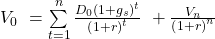The first term is discounting the dividends during the high growth period. The second term is calculating the terminal value for the second sustainable growth period and then discounting it to the present value where Vn = terminal value at time n estimated using the Gordon growth model. Example Let us understand the concept better with the help of an example. The current dividend for a company is$4.00. The dividends are expected to grow at 20% a year for 4 years and then at 10%  after that. The required rate of return is 18%. Estimate the intrinsic value.

Solution:

First draw a timeline.

We will use this formula:where n = 4 (high growth period)

Solve for the second term: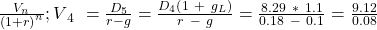= 114

Using the financial calculator, we can calculate the present value of dividends and terminal value by entering the following values: CF= 0; CF= 4.8; CF= 5.76; CF= 6.91; CF= 8.29 + 114; I = 18; NPV = 75.48

Note: while calculating V4, you need to use 10% as growth rate since it is the long term growth rate.

Three Stage Models

The concept of a two-stage model can be extended to as many stages as a company goes through. Often, companies go through three stages beyond the startup phase: growth, transition and maturity.

LIVE WEEKEND CLASSES *For 2024 Exam candidates*
This is default text for notification bar